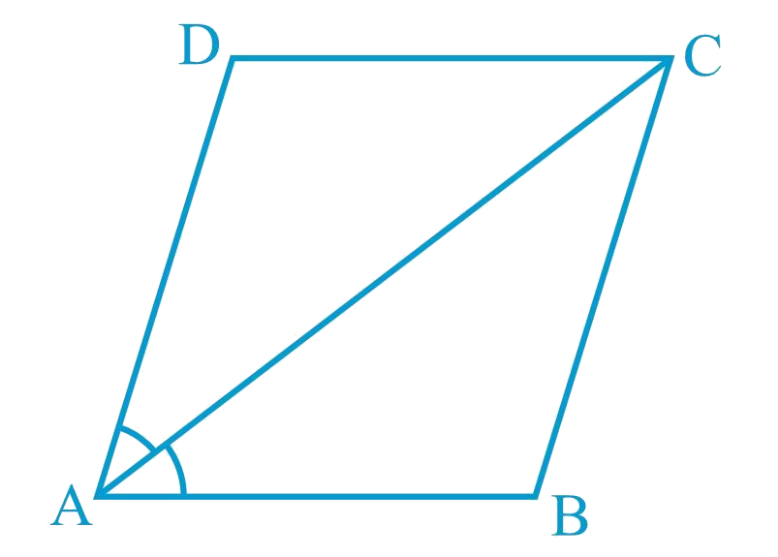In the verge of coronavirus pandemic, we are providing FREE access to our entire Online Curriculum to ensure Learning Doesn't STOP!

# Ex.8.1 Q6 Quadrilaterals Solution - NCERT Maths Class 9

Go back to  'Ex.8.1'

## Question

Diagonal $$AC$$ of a parallelogram $$ABCD$$ bisects $$\angle A$$ (see the given figure). Show that

(i) It bisects $$\angle C$$ also,

(ii) $$ABCD$$ is a rhombus.

Video Solution
Ex 8.1 | Question 6

## Text Solution

What is known?

The diagonal AC of a parallelogram $$ABCD$$ bisects $$∠A.$$

What is unknown?

How we can show that (i) It bisects $$∠C$$ also, (ii) ABCD is a rhombus.

Reasoning:

We can use alternate interior angles property to show diagonal $$AC$$ bisects angle $$C$$ also, by showing all sides equal it can be said rhombus.Steps:

(i) $$ABCD$$ is a parallelogram.

\begin{align} &\angle \mathrm{DAC}=\angle {BCA}\\&\text{(Alternate interior angles are equal)} \ldots(1)\end{align}

\begin{align}\text{And,} &\angle \mathrm{BAC}=\angle {DCA}\\&\text{(Alternate interior angles are equal)} \ldots(2)\end{align}

However, it is given that $$AC$$ bisects $$\angle A$$.

$$\angle \mathrm{DAC}=\angle \mathrm{BAC} \ldots(3)$$

From Equations ($$1$$),($$2$$), and ($$3$$), we obtain

\begin{align} {\angle D A C}&\!=\!\!{\angle B C A\!=\!\!\angle B A C\!=\!\!\angle D C A \ldots\!(\!4\!)} \\ {\angle D C A}&\!=\!\!{\angle B C A}\end{align}

Hence, $$AC$$ bisects $$\angle C$$.

(ii) From Equation ($$4$$), we obtain

$$\angle {DAC}=\angle {DCA}$$

$${{DA}}={DC}$$(Side opposite to equal angles are equal)

However, $$DA = BC$$ and $$AB = CD$$

(Opposite sides of a parallelogram)

$$AB = BC = CD = DA$$

Hence, $$ABCD$$ is a rhombus.

Video Solution